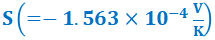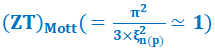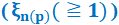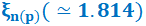, same minimum Seebeck coefficient, and same"> , same minimum Seebeck coefficient, and same"> , same minimum Seebeck coefficient, and same">Volume 8, Number 4 (2023)
Year Launched: 2016
ISSN: 2706-8862
Previous Issues
Why Us
-  Open Access
-  Peer-reviewed
-  Rapid publication
-  Free indexing service
-  Free promotion service
-  More citations
-  Search engine friendly
Email:   service@scirea.org
1 Rockefeller Plaza, 10th and 11th Floors, New York, NY 10020 U.S.A.

# Same maximum figure of merit ZT(=1), due to the effect of impurity size, obtained in the n(p)-type degenerate Ge-crystal, at same reduced Fermi energy, same minimum Seebeck coefficient, and sameVolume 8, Issue 4, August 2023    |    PP. 407-430    |PDF (1437 K)|    Pub. Date: August 8, 2023

Author(s)
H. Van Cong, Université de Perpignan Via Domitia, Laboratoire de Mathématiques et Physique (LAMPS), EA 4217, Département de Physique, 52, Avenue Paul Alduy, F-66 860 Perpignan, France.

Abstract
In our two previous papers [1, 2], referred to as I and II. In I, our new expression for the extrinsic static dielectric constant, \varepsilon\left(r_{d\left(a\right)}\right), r_{d\left(a\right)} being the donor (acceptor) d(a)-radius, was determined by using an effective Bohr model, suggesting that, for an increasing r_{d\left(a\right)}, \varepsilon\left(r_{d\left(a\right)}\right), due to such the impurity size effect, decreases, and affecting strongly the critical impurity density in the metal-insulator transition and also various majority carrier transport coefficients given in the n(p)-type degenerate Ge -crystal, defined for the reduced Fermi energy \mathbf{\xi}_{\mathbf{n}(\mathbf{p})}(\geqq\mathbf{1}). Then, using the same physical model and same mathematical methods and taking into account the corrected values of energy-band-structure parameters, all the numerical results, obtained in II, are now revised and performed, giving rise to some important concluding remarks as follows.(1) The critical donor (acceptor)-density, N_{CDn\left(NDp\right)}(r_{d(a)}), determined in Eq. (3), can be explained by the densities of electrons (holes) localized in exponential conduction (valance)-band (EBT) tails, N_{CDn\left(CDp\right)}^{EBT}(r_{d(a)}), given in Eq. (21). (2) In Tables 9-10, with a given d(a)-density N [=2\timesN_{CDn\left(NDp\right)}(r_{d(a)})], one notes here that with increasing temperature T(K): (i) for reduced Fermi energy \xi_{n(p)}(\simeq1.814), while the numerical results of the Seebeck coefficient S present a same minimum \left(=-1.563\times{10}^{-4}\frac{V}{K}\right), those of the figure of merit ZT show a same maximum ZT\left(=\mathbf{1}\right), (ii) for \xi_{n(p)}=1, those of S and ZT present same results: Sb\left(=-1.322\times{10}^{-4}\frac{V}{K}\right) and 0.715, respectively, (iii) for \xi_{n(p)}\simeq1.814 and \xi_{n(p)}=1, the same values of the well-known Mott figure of merit, \left(ZT\right)_{Mott}=\frac{\pi^2}{3\times\xi_{n(p)}^2}(\simeq1 and 3.29), are respectively investigated, and finally, (iv) we show here that in the degenerate Ge-semiconductor, the Wiedemann-Frank law, given in Eq. (25a), is found to be exact.

Keywords
Effects of the impurity-size and heavy doping; effective autocorrelation function for potential fluctuations; optical, electrical, and thermoelectric properties; figure of merit; Wiedemann-Franz law

Cite this paper
H. Van Cong, Same maximum figure of merit ZT(=1), due to the effect of impurity size, obtained in the n(p)-type degenerate Ge-crystal, at same reduced Fermi energy, same minimum Seebeck coefficient, and same, SCIREA Journal of Physics. Vol. 8 , No. 4 , 2023 , pp. 407 - 430 . https://doi.org/10.54647/physics140568

Most Views
Article
by Sergey M. Afonin
Article
by Jian-Qiang Wang, Chen-Xi Wang, Jian-Guo Wang, HRSCNP Research Team
Article
by Sergey M. Afonin
Article
by Alvaro Valdivia Pareja, Diana Stark Ekman
Article
by Syed Adil Hussain, Taha Hasan Associate Professor